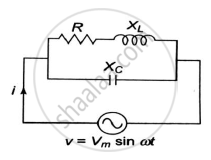# Derive the Formula for Resonant Frequency of the Circuit with a Pure Capacitor in Parallel with a Coil Having Resistance and Inductance. - Basic Electrical and Electronics Engineering

Sum

Derive the formula for resonant frequency of the circuit with a pure capacitor in parallel with a coil having resistance and inductance. Find the expression for dynamic resistance of this parallel resonant circuit.

#### SolutionConsider a parallel circuit consisting of a coil and a capacitor as shown below. The impedances of two branches are:-

overline(Z_1)=R+jX_L       overlineZ_2=-jX_c

overlineY_1=1/Z_1=1/(R+jX_L)=(R-jX_L)/(R^2+X_L^2)      overline(Y_2)=1/overline(Z_2)=1/(-jX_C)=j/X_C

Admittance of the circuit overline(Y)=overline(Y_1)+overline(Y_2)

overline(Y)=(R-jX_L)/(R^2+X_L^2)+j/X_C   =  R/(R^2+X_L^2)-j(X_L/(R^2+X_L^2)-1/X_C)

At resonance the circuit is purely resistive. Therefore, the condition for resonance is.

X_L/(R^2+X_L^2)-1/X_C=0

X_L/(R^2+X_L^2)=1/X_C

X_LX_C=R^2+X_L^2

omega_oL 1/(omega_0C)=R^2+omega_0^2L^2

omega_0^2L^2=L/C-R^2

omega_0=sqrt(1/(LC)-R^2/L^2)

f_0=1/(2pi)sqrt(1/(LC)-R^2/L^2)

Where 𝑓0 is called as the resonant frequency of the circuit.
If R is very small as compared to L then

omega_0=sqrt1/(LC)

f_0=1/(2pisqrt(LC))

DYNAMIC IMPEDANCE OF A PARALLEL CIRCUIT.

At resonance the circuit is purely resistive the real part of admittance is R/(R^2+X_L^2).Hence the dynamic impedance at resonance is given by,

Z_D=(R^2+X_L^2)/R\

At resonance ,

R^2+X_L^2=X_LX_C=L/C

Z_D=L/(CR)

Concept: Series and Parallel Resonance
Is there an error in this question or solution?